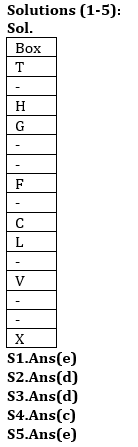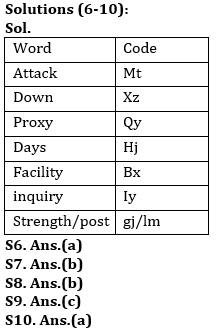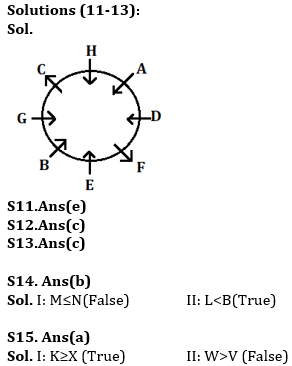Latest Banking jobs   »   Reasoning Ability Quiz For ESIC- UDC,...

# Reasoning Ability Quiz For ESIC- UDC, Steno, MTS Prelims 2022- 10th January

Directions (1-5): Study the information carefully and answer the questions given below.
A certain number of boxes are placed in a stack one above other. Box H is placed just above box G. Only two boxes are placed between G and F. Four boxes are placed between F and V. Only two boxes are placed between V and X, which is below box V. As many boxes between box H and box C as many boxes are placed between C and X. More than twelve boxes are placed between the box which is placed bottom most and T. Not more than one box placed between Box H and box T. box T placed topmost of stack. Two boxes are placed between box F and L.

Q1. How many boxes are placed in the stack?
(a) Twelve
(b) Thirteen
(c) Eleven
(d) Fourteen
(e) None of these

Q2. Which of the following box is placed just below box C?
(a) V
(b) F
(c) H
(d) L
(e) None of these

Q3.How many boxes are placed between box V and H?
(a) Six
(b) Seven
(c) Four
(d) More than seven
(e) None

Q4. If box D is placed just below box G then how many boxes are placed between Box T and Box D?
(a) Two
(b) Four
(c) Three
(d) None
(e) One

Q5. Which of the following box is placed at bottom of stack?
(a) V
(b) L
(c) G
(d) H
(e) X

Directions (6-10): Study the following information carefully and answer the questions given below:
In a certain code language,
‘attack proxy down’ is written as ‘xz mt qy’,
‘proxy days facility’ is written as ‘qy hj bx’,
‘post strength attack’ is written as ‘lm mt gj’.
‘down days inquiry’ is written as ‘xz hj iy’,

Q6. What is the code for ‘facility’?
(a) bx
(b) hj
(c) qy
(d) lm
(e) None of these

Q7. Which of the following may be the code for ‘strength down’?
(a) gj hj
(b) lm xz
(c) bx lm
(d) gj iy
(e) None of these

Q8. What is the code for ‘Inquiry’?
(a) hj
(b) iy
(c) xz
(d) lm
(e) Can’t be determined

Q9. What does ‘xz’ stand for?
(a) Post
(b) Strength
(c) Down
(d) days
(e) None of these

Q10. What is the code for ‘Proxy’?
(a) qy
(b) hj
(c) bx
(d) mt
(e)None of these

Directions (11-13): Study the information carefully and answer the questions given below.
Eight persons are sitting in a circular table in such a way that some are facing towards center and some are facing outside the center. D sits second to the right of E, who faces towards the center. B sits third to the left of D. C sits opposite to F. One person sits between H and G. H and C sits immediate right to each other’s. H does not sit opposite to the one who sits immediate left of A. A faces towards center. B and G face same direction to H. F face same direction to C. C does not sit immediate left of E.

Q11. Who among the following sits second to the left of A?
(a) D
(b) G
(c) B
(d) H
(e) None of these

Q12. How many persons sit between C and E, when counted to the left of C?
(a) Three
(b) One
(c) Two
(d) Four
(e) None

Q13. How is B related to H?
(a) Second to the left
(b) Immediate right
(c) Third to the right
(d) Fourth to the left
(e) None of these

Directions (14-15): In these questions, relationship between different elements is show in the statements. The statements are followed by conclusions. Study the conclusions based on the given statements and select the appropriate answer:
(a) If only conclusion I follows.
(b) If only conclusion II follows.
(c) If either conclusion I or II follows
(d) If neither conclusion I nor II follows.
(e) If both conclusions I and II follow.

Q14. Statements: A≤M<T≤B=N>R>Y≥P>L
Conclusion I: M≤N
II: L<B

Q15. Statements: K≥V=E≥M=X≤W>L≥S
Conclusion I: K≥X
II: W>V

Solutions#### Congratulations!Union Budget 2023-24: Free PDF